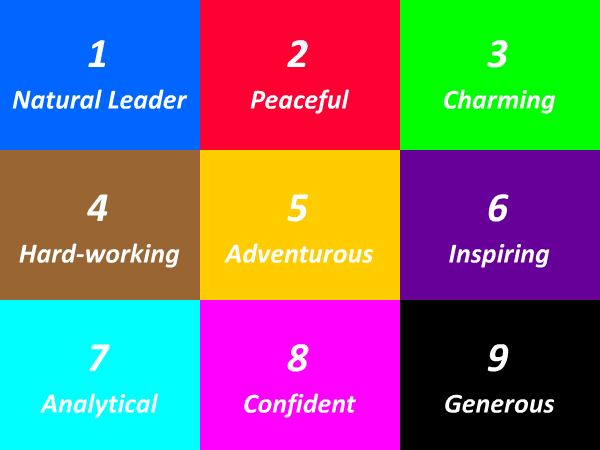# 100 Times Table - Generate Multiplication Table of 100

100 Times Table
1x100=100
2x100=200
3x100=300
4x100=400
5x100=500
6x100=600
7x100=700
8x100=800
9x100=900
10x100=1000
11x100=1100
12x100=1200
13x100=1300
14x100=1400
15x100=1500
16x100=1600
17x100=1700
18x100=1800
19x100=1900
20x100=2000
21x100=2100
22x100=2200
23x100=2300
24x100=2400
25x100=2500
100 Times Table
26x100=2600
27x100=2700
28x100=2800
29x100=2900
30x100=3000
31x100=3100
32x100=3200
33x100=3300
34x100=3400
35x100=3500
36x100=3600
37x100=3700
38x100=3800
39x100=3900
40x100=4000
41x100=4100
42x100=4200
43x100=4300
44x100=4400
45x100=4500
46x100=4600
47x100=4700
48x100=4800
49x100=4900
50x100=5000
100 Times Table
51x100=5100
52x100=5200
53x100=5300
54x100=5400
55x100=5500
56x100=5600
57x100=5700
58x100=5800
59x100=5900
60x100=6000
61x100=6100
62x100=6200
63x100=6300
64x100=6400
65x100=6500
66x100=6600
67x100=6700
68x100=6800
69x100=6900
70x100=7000
71x100=7100
72x100=7200
73x100=7300
74x100=7400
75x100=7500

## Test Your Skills Here

The 95 times table quiz is very useful in the improvement of kid's multiplication and maths skills. Here you will find a selection of times table tests designed to help your child to learn and practice their times tables. Fill in your answers. Once you have entered all the answers, click on 'Check' button to see whether you have got them all correct. If your answers is Wrong then we will show you the 'Correct' answer.

Practice Excersise
95 x 18 =
95 x 18 =
95 x 12 =
95 x 11 =
95 x = 1045
95 x = 2850
95 x = 1140
95 x 16 =
95 x = 1140
95 x = 2755#### More Tables#### Top Search Quesries for this page:

• 95 times table
• 95 times tables
• table of 95
• 95 multiplication table
• multiplication chart 95
• multiplication of 95
• 95 table maths### Spreading Knowledge Across the World

USA - United States of America  Canada  United Kingdom  Australia  New Zealand  South America  Brazil  Portugal  Netherland  South Africa  Ethiopia  Zambia  Singapore  Malaysia  India  China  UAE - Saudi Arabia  Qatar  Oman  Kuwait  Bahrain  Dubai  Israil  England  Scotland  Norway  Ireland  Denmark  France  Spain  Poland  and  many more....